I.5.9

8/20/2021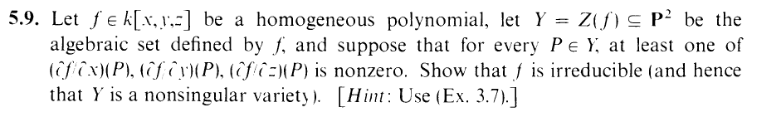Break up f into its irreducible components:

 f = f1ft

which likewise breaks Y into its irreducible components:

 Y = Z(f1) ∪∪ Z(ft)

Let P Z(f1) Z(f2), and assume without loss of generality that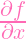(P)0 (as given by the condition). Note that, using the product rule,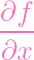=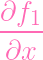⋅ f2ft +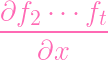⋅ f1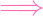(P) =(P) ⋅ f2(P)ft(P) +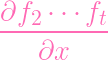(P) ⋅ f1(P) = 0 (since f1(P) = 0,f2(P) = 0

But this contradicts the nonzeroness of the partial at P. Hence Z(f1) Z(f2) = . But this contradicts 3.7. Hence Y must only have one irreducible component i.e. Y is irreducible (i.e. f is irreducible). Now that we know Y is a projective variety, we can apply what we learned yesterday ("Paddle Person"... seriously? Fuck my life. I deserve to die). Since the gradient vector (Jacobian) has a nonzero partial derivative at every point, we're dun.